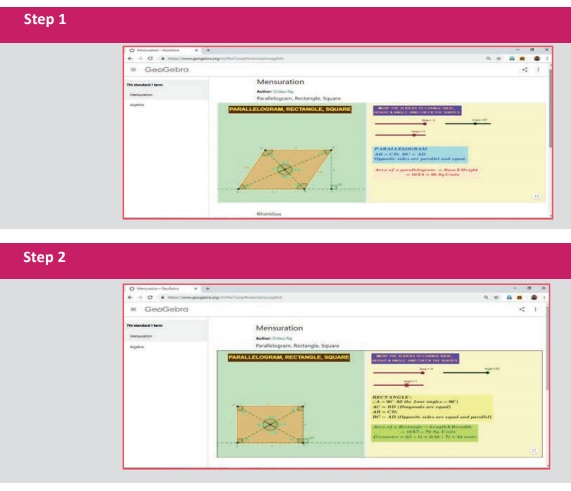Home | | Maths 7th Std | Summary

# Summary

7th Maths : Term 1 Unit 2 : Measurements : Summary

Summary

● Parallelogram is a four sided closed shape in which opposite sides are both parallel and equal.

Area of the Parallelogram = b × h sq. units, where b = base ; h = height.

● In a parallelogram if all the sides are equal then it is called Rhombus.

● Area of rhombus = 1/2 × d1 × d2 sq. units, where d1, d2 are the diagonals.

● A parallelogram with one pair of non-parallel sides is known as a Trapezium.

● Area of Trapezium = 1/2 × h(a+b) sq. units

● If the non - parallel sides of a Trapezium are equal then it is known as an isosceles Trapezium.

ICT Corner

Expected Result is shown in this pictureStep – 1 :

Open the Browser type the URL Link given below (or) Scan the QR Code. GeoGebra work sheet named “7th std Mensuration” will open. In the right side of the work sheet there are three sliders to change Length, Breadth and Corner angle.

Step - 2 :

Move the sliders to get, Parallelogram or Rectangle or Square and see the points given and observe.Mensuration: https://ggbm.at/zzvqg9vh

or Scan the QR Code.

Tags : Measurements | Term 1 Chapter 2 | 7th Maths , 7th Maths : Term 1 Unit 2 : Measurements
Study Material, Lecturing Notes, Assignment, Reference, Wiki description explanation, brief detail
7th Maths : Term 1 Unit 2 : Measurements : Summary | Measurements | Term 1 Chapter 2 | 7th Maths